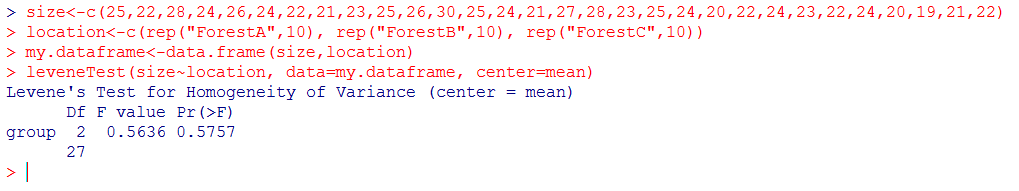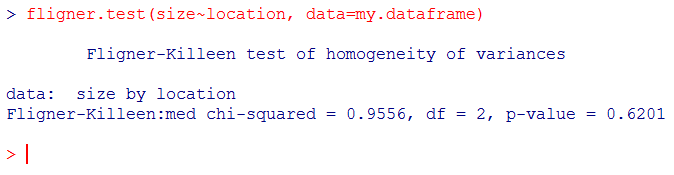# Test for homogeneity of variances – Levene’s test and the Fligner Killeen test

Several tests such as Student’s t-test and ANOVA require that the groups to compare have equal variances. Testing for homogeneity of variances in R is rather easy and several functions may be used, depending on a couple of factors.

2 tests are commonly used to perform such an analysis: Fisher’s F test and Levene’s test. While the first one, which we have already introduced here, is restricted to comparison of two variances/groups (and is therefore useful when performing a t-test), the second one is used in connection to ANOVA where more than two groups must be compared.

Levene’s test checks for homogeneity of variances and the null hypothesis is that all variances are equal. A resulting p-value under 0.05 means that variances are not equal and than further parametric tests such as ANOVA are not suited. Note that this test is meant to be used with normally distributed data but can tolerate relatively low deviation from normality.

The corresponding function in R is `leveneTest(dataset~groups, data=dataframe)` where `dataset` is the vector containing the numerical data, `groups` is the vector that contains the names/labels of the groups to compare and `data=` is followed by the name of the whole dataframe containing `dataset` and `groups`. The function is `leveneTest()` found in the pre-installed package `car `in R. To activate `car`,  simply type in the following line:

```library(car)
```

To introduce Levene’s test, we use (or reuse) an example which is presented in the article on one-way ANOVA. We build the dataframe my.dataframe with the vector size and location and run the test:

```size<-c(25,22,28,24,26,24,22,21,23,25,26,30,25,24,21,27,28,23,25,24,20,22,24,23,22,24,20,19,21,22)
location<-c(rep("ForestA",10), rep("ForestB",10), rep("ForestC",10))
my.dataframe<-data.frame(size,location)
leveneTest(size~location, data=my.dataframe, center=mean)
```And the test reveals a p-value greater than 0.05, indicating that there is no significant difference in variances between the groups in `location`. Note that we have added a parameter in the above-mentioned function, namely `center=mean`, which tells R to consider the mean of each group in the calculations. By default, `leveneTest()` uses the median as center (`center=median`), which renders the test more robust. In that case the true name of the test is Brown-Forsythe test for homogeneity of variance.

The Fligner-Killeen test does a rather similar job, meaning that it checks for homogeneity of variance, but is a much better option when data are non-normally distributed or when problems related to outliers in the dataset cannot be resolved.

The function is `fligner.test(dataset~groups, data=dataframe)` which is very similar in syntax to `leveneTest( ).`

```fligner.test(size~location, data=my.dataframe)
```and again the p-value is high enough to let you know that variances are homogeneous.

Fant du det du lette etter? Did you find this helpful?
[Average: 5]